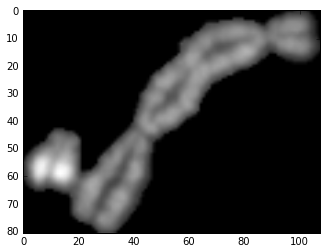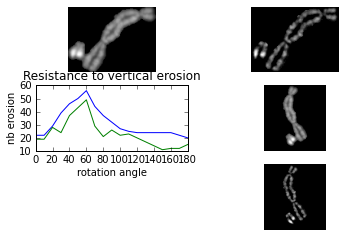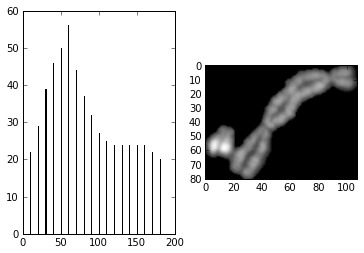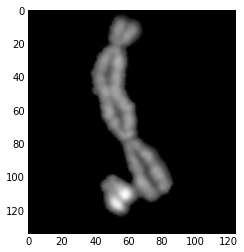In :
from __future__ import division
import numpy as np
from scipy import ndimage as nd
import pymorph
import mahotas
import mahotas.polygon
import pylab
import os

In :
# -*- coding: utf-8 -*-
"""
Created on Thu Apr  7 15:19:19 2011

@author: Jean-Patrick Pommier
http://www.dip4fish.blogspot.com
"""

def HighPass(im,size):
blur=nd.gaussian_filter(im, size)
hi=pymorph.subm(im,blur)
return hi

def PeakByModalValue(array):
'''look for the modal value of a 2D array'''
x=array[0,:]
y=array[1,:]
print "y.shape",y.shape
print "y",y
print "y[y.shape-1]",y[y.shape-1]
print "Searching modal value"
xmin=x.min()#image min graylevel
xmax=x.max()#image max gray level
mode=xmin
countmax=0#occurence of a given grayscale
#print "mig=",xmin,"  mag=",xmax
for i in range(0,y.shape-1):
test=y[i]>countmax
#print "test:",test,"histo(",i,")=", y[i],"max",countmax
if  test:
countmax=y[i]
mode=x[i]
#print "mode",mode
return mode

class particle(object):
rotatedIm=np.array([[0,0],[0,0]])
rotatedFlag=False
#ratio of particle area by convexhull area
#close to 1 for a convex particle
#lower for other as touching chromosomes
CvxhParticleArea_ratio=0

def __init__(self,arrayIm):
'''
Initially the rotated image is empty and the flag
indicates that no rotation is performed
'''
self.particuleImage=arrayIm
self.rotatedIm=np.array([[0,0],[0,0]])
self.compassTable=np.array([[0,0],[0,0]])
self.compassTablePeaks=np.array([[0,0],[0,0]])

def cvxhull_area(self):
'''
Calculate the convexhull area such that:
A=0.5*Sumfrom 0 to N-1 of {xn+1*yn-xn*yn+1}
Pn(xn,yn) and PN=P0
see http://en.wikipedia.org/wiki/Polygon#Area_and_centroid
'''
#print "cvxhull called"
binIm=self.particuleImage>0
area=np.sum(binIm[:,:]==True)
print "area",area
#print binIm.dtype
contour=mahotas.bwperim(binIm)
#print "contour",contour.dtype
pointlist=mahotas.polygon.convexhull(contour)
N=len(pointlist)
fP=pointlist
#duplicate the first point P0
#at the end such that PointN=Point0
pointlist.append(fP)
s=0
#compute the sum from 0 to N-1
for i in range(0,N-1):
cx=pointlist[i]#x of the current point
cy=pointlist[i]#y of the current point
#print "Point",i," x=",cx," y=",cy
nx=pointlist[i+1]#x of the next point
ny=pointlist[i+1]#y of the next point
#print "Point suiv",i+1," x=",nx," y=",ny
det=nx*cy-cx*ny
s=s+det
#print "det:",det," S=",s
CvxhParticleArea_ratio=area/(0.5*abs(s))
return 0.5*abs(s),CvxhParticleArea_ratio

def getVerticalImage(self):
return self.rotatedIm

def getcompassTableDerivative(self):
return self.compassTablePeaks

def orientationByErosion(self,step):
''' Performs successive rotations of a binary particle from 0 to 180 by "step"
Then at each angle performs:
*successives erosions
*pixel counts
Find relationship between angle and the minimal erosion to destroy the particle
An anisotropic particle such a long chromosome should have a
principal orientation, overlapping chromosomes should have at least two
orientation.
'''
def compassTableDerivative(table):
deriv=np.zeros(table.shape)
x=table[0,:]
y=table[1,:]
for i in range(1,y.shape-1):
deriv[1,i]=(y[i+1]-y[i])/((x[i+1]-x[i]))
deriv[0,i]=x[i]
print "delta y",y[i+1]-y[i]
print "delta x",x[i+1]-x[i]
print "der",deriv[1,i]
self.compassTablePeaks=np.copy(deriv)
return self.compassTablePeaks

def erodeVParticle(im):
'''Counts the number of vertical erosion neccessary
to destroy a particule
vline is a 3X1 structuring element
DestroyParticle returns the scalar number:nb_erosion
necessary to erode totally a binary particle'''

def countTruePix(bim):
'''bim must be a flat binary (True,False) array
counts the occurence of True (pixel) in a binary array/image '''
return np.sum(bim[:,:]==True)
vline=np.array([[0,1,0],
[0,1,0],
[0,1,0]])
#threshold the image
binaryIm=(im>0)
pixcount=countTruePix(binaryIm)
nb_erosion=1
while pixcount>0:
erodedIm=nd.binary_erosion(binaryIm,structure=vline,iterations=nb_erosion)
nb_erosion=nb_erosion+1
pixcount=countTruePix(erodedIm)
#erodedParticle work should be done here
return nb_erosion
#
#Analysis of the particle orientation starts here
#
maxRotationNubr=180/step
#rotation angle from 0 to 180 by step of 5°
compassTable=np.zeros((2,maxRotationNubr+1),dtype=np.uint8)
#Store
#print compassTable.shape
i=0
angle=i*step
rotatedIm=self.particuleImage
#print self.particuleImage.shape
while i<=maxRotationNubr:
maxErosion=erodeVParticle(rotatedIm)
compassTable[0,i]=angle
compassTable[1,i]=maxErosion
#print "i=",i," angle=",angle," -erosion max:",maxErosion
rotatedIm=nd.rotate(self.particuleImage,angle)
i=i+1
angle=i*step
majorAngle=PeakByModalValue(compassTable)
self.rotatedIm=nd.rotate(self.particuleImage,majorAngle)
self.rotatedFlag=True
return majorAngle,compassTable,self.rotatedIm


In :
import urllib

image_name = "part14.png"
web = urllib.URLopener()
web.retrieve(url, image_name)
imshow(image[:,:,0])

Out:
<matplotlib.image.AxesImage at 0xb8e720c>In :
im=image[:,:,0]
hip=pymorph.subm(im,nd.gaussian_filter(im,3))
#print im.dtype#print uint16
p1=particle(im)
#print "particle 1",p1.cvxhull_area()
p2=particle(hip)
#print "particle 1 hi pass",p2.cvxhull_area()
theta1,rosedesvents,VImage=p1.orientationByErosion(10)
theta2,rdv,VHip=p2.orientationByErosion(10)
x=rosedesvents[0,:]
y=rosedesvents[1,:]
xh=rdv[0,:]
yh=rdv[1,:]

y.shape 19
y 22
y[y.shape-1] 20
Searching modal value
y.shape 19
y 19
y[y.shape-1] 15
Searching modal value

In :
pylab.subplot(321,frameon=False, xticks=[], yticks=[])
pylab.gray()
pylab.imshow(im)

pylab.subplot(322,frameon=False, xticks=[], yticks=[])
pylab.imshow(hip)

pylab.subplot(323)
pylab.title("Resistance to vertical erosion")
pylab.ylabel("nb erosion")
pylab.xlabel("rotation angle")
pylab.plot(x,y,xh,yh)

pylab.subplot(324,frameon=False, xticks=[], yticks=[])
pylab.imshow(VImage)

pylab.subplot(326,frameon=False, xticks=[], yticks=[])
pylab.imshow(VHip)
pylab.show()In :
subplot(121, polar=False)
bar(x, y, width=0.1, bottom=0.0)

subplot(122, polar=False)
imshow(im)

show()In :
vert = p1.getVerticalImage()
imshow(vert)
show()In :


In [ ]: